# Clustering

Note that adaptive binning will take a set of events, create a histogram on every parameter being clustered for those events, and then examine if any of those histograms could be divided usefully. The following parameters control how this histogram examination is performed to divide events further and further into smaller and smaller bins.

Minimum Separation Channel: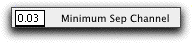Clustering will not divide any population below this fraction of the total scale of a parameter. Thus, a value of "0.25" means don't divide any subset within the first decade (on a 4-decade log scale). Setting this value too high will not allow the platform to resolve populations near the low end of the scale. Too low, and it may create "biologically-irrelevant" populations.

Range of values: 0 - 1
Default value: 0.03 (roughly, 1/8th of a decade in a 4-decade scale).
Other useful values: 0.06, 0.125, 0.25

Histogram Resolution:The number of channels used for histogramming. Channels are equally spaced (linear parameter), or log-spaced (log parameters), exactly as for creating displays. Setting this value too high could cause subsets to be "orphaned" during the "shaving" of empty space off of histograms during adaptive binning... this could lead to clusters that cannot be joined. In addition, the clustering algorithm will take longer with larger values. Setting this value too low will lead to an inability to distinguish clusters.

Range of values: None (However, less than 16 is probably useless, as is more than 1024)
Default value: 256.
Other useful values: 128, 64.

Minimum Shave Fraction: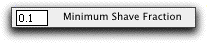During adaptive binning, one major criterion for dividing a distribution into bins is whether or not there is "empty" space at one end of the histogram. Such empty space will be "shaved off" into a separate bin from the data. Since bins that are not physically adjacent can never be re-joined into a cluster, shaving too aggressively can lead to the inability to rejoin event-containing bins into real clusters. For this clustering algorithm, the shaving step is one of the most critical.

This value specifies how much of a distribution in a given parameter must be devoid of events before the platform considers "shaving" it off. Thus, a value of "0.1" means that 10% of the distribution's width (either upper or lower end) must be completely devoid of events before shaving is considered. A value that is too small will lead to too many orphaned clusters. A value that is too large will not allow sufficient resolution to segregate otherwise close clusters.

Range of values: 0 - 1
Default value: 0.1.

Minimum Shave Channels: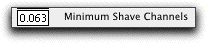See above discussion on "Minimum Shave Fraction." In addition to that criterion, the platform will never shave off any empty space if it contains less than a fixed number of channels, which is this value times the histogram resolution. In other words, this value defines the smallest empty space that can be created during binning.

Range of values: 0 - 1
Default value: 0.063 (approximately 1/4th decade on a log scale)
Other useful values: Smaller, probably as low as 0.01 might be useful.

Maximum Value Height Ratio:During adaptive binning, the other criterion for dividing a distribution that is always checked is whether or not there is a bimodal distribution. This value determines what kind of distribution is considered bimodal. If a valley exists between two peaks, then the distribution is bimodal. A valley must be no higher than this value times the lower of the two peaks. (In other words, a value of 0.5 means that the valley must be no higher than half of the height of the lower peak). If there is no point in the distribution where this exists, then the distribution is not considered bimodal. A value that is too small will prevent the platform from finding useful peaks in a distribution. A value that is too high will divide events seemingly randomly.

Range of values: 0 - 1
Default value: 0.5
Useful value: 0.75

Separation: Valley Weighting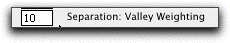Separation: Even Division Weighting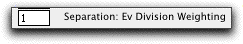If, for any given set of events, there is more than one parameter that is bimodal, then these two values determine which one better separates the events. Since adaptive binning is iterative, it probably doesn't matter which parameter is first divided; the other will be divided shortly. Nonetheless, these two parameters provide the relative weighting to compare two such divisions. A larger value on valley weighting means that emphasis is place more on how deep a valley is between the two peaks. A larger value on the "Ev Division" means that emphasis is placed on divisions that more evenly divide the events by number.

Range of values: 0 - infinity
Default values: 1 and 10
Useful values: 10 and 1

Allow division of uniform clusters
If set, then adaptive binning is instructed to divide a uniform cluster of events. This occurs when no shaving can occur and no "valley" between peaks can be found in any parameter. If this option is selected, then Cluster Joining will be required.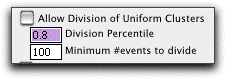Division percentile
If the platform decides to divide a uniform cluster, then it will do so at this percentile of the distribution. A value of 0.5 means to divide the distribution evenly. A value of 0.8 means to divide the cluster at either the 20th or the 80th percentile, whichever gives a larger division in terms of area. Values further from 0.5 will tend to create more clusters near edges of event densities.

Range of values: 0 - 1
Default value: 0.8
Useful values: 0.5, 0.9

Minimum # of events to divide
If the number of events in a uniform cluster is less than this value, then the cluster is not divided. In general, if the uniform division is allowed, then there will be no cluster with more than this number of events. Therefore, this number effectively determines a lower bound on the number of clusters. A small value will therefore create many more clusters, and require correspondingly much greater computational time at joining.

Range of values: 1 - infinity
Default value: 100
Useful values: about 0.1 to 1.0% of the number of events in the file

Do simple Peak Find Separation
If selected, then the platform will attempt a more sophisticated peak finding algorithm to find populations (i.e., more sophisticated than the valley search performed by default). This algorithm might allow for the identification and separation of "shoulder" clusters. The algorithm functions by scanning on either side of the mode of a histogram. As it moves along either side, it computes the local slope of the histogram. By comparing the Based on the following parameters, a determination is made as to whether or not a peak has been found, and if so, what its extents are.

Chan Width of Slope Function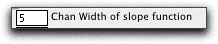The number of channels over which a running slope is calculated. A large value will, in effect, use a heavily smoothed histogram to look for a peak. A small value will obviate the utility of the peak find.

Range of values: 1 - infinity
Default value: 5

Minimum Peak Height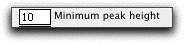A peak must be at least this many cells in a single channel before it is considered a true "peak". Large values will tend to prevent the algorithm from finding a peak; small values will tend to identify too many peaks.

Range of values: 1 - infinity
Default value: 10

Trigger Slope: Mode Ratio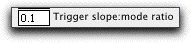A peak has been found when local slope (per channel) is more than this factor times the mode value.

Range of values: 1 - infinity
Default value: 0.1
Useful values: 0.01 - 0.5

Fire slope Ratio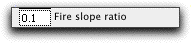The end of the peak is identified when the local slope is less than this factor times the maximum slope that occurred since the mode. i.e., a value of 0.1 means that the farthest reaches of the peak occurs when the slope is only 10% (or less) of the maximum slope between this point and the mode. Larger values will find more subtle shoulders in a distribution, but are more prone to simply randomly dividing the distribution. A value of 0 would require a true "valley"--i.e, for the distribution to start rising again.

Range of values: 0 - 1
Default value: 0.1

Joining Criteria:
If joining is initiated, then the algorithm attempts to join all physically adjacent bins into clusters. Bins that are not physically adjacent (tangent) are not considered; hence, the "shaving" function above cannot be too aggressive.Minimum
Joining ceases when this many clusters remain. Since the order in which bins are joined is not well-defined, it is unlikely that this value should ever be changed from the default of 1.

Max InterClus Dist x
In order for two clusters to be joined, not only must they be tangent but the distributions of each cluster must not be significantly changed by the join. Currently, this is tested as making sure that for every parameter involved in the cluster, the distance between the centers of the two clusters is no more than this value times the width of each cluster. Therefore, larger values will tend to join clusters that are more distinct; smaller values will tend to keep clusters apart.

Range of values: 0 - infinity.
Default value: 2
Useful values: 1 - 3.